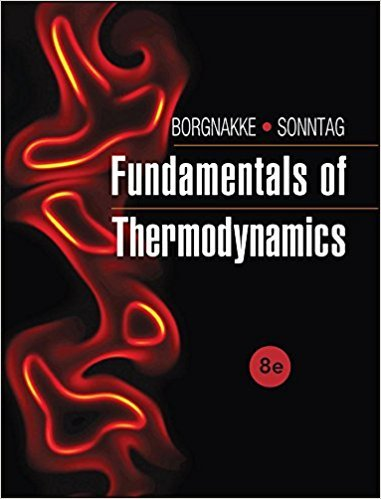×
Get Full Access to Fundamentals Of Thermodynamcs - 8 Edition - Chapter 3 - Problem 94hp
Get Full Access to Fundamentals Of Thermodynamcs - 8 Edition - Chapter 3 - Problem 94hp

×

# A piston/cylinder contains air at 600 kPa, 290 K and aISBN: 9781118131992 58

## Solution for problem 94HP Chapter 3

Fundamentals of Thermodynamcs | 8th Edition

• Textbook Solutions
• 2901 Step-by-step solutions solved by professors and subject experts
• Get 24/7 help from StudySoup virtual teaching assistantsFundamentals of Thermodynamcs | 8th Edition

4 5 1 343 Reviews
31
4
Problem 94HP

Problem 94HP

A piston/cylinder contains air at 600 kPa, 290 K and a volume of 0.01 m3. A constant-pressure process gives 54 kJ of work out. Find the final volume, the temperature of the air, and the heat transfer.

Step-by-Step Solution:

Solution 94HP

Step 1 of 6

In the given case we have to calculate the final volume, final temperature of air and the heat transfer during the constant pressure process.

Given data,

Pressure,Initial temperature of air,Initial volume of air,Work done,To find,

Final temperature of air,Final volume of air,Heat transfer,Step 2 of 6

Step 3 of 6

##### ISBN: 9781118131992

The full step-by-step solution to problem: 94HP from chapter: 3 was answered by , our top Engineering and Tech solution expert on 08/03/17, 05:05AM. Since the solution to 94HP from 3 chapter was answered, more than 1194 students have viewed the full step-by-step answer. Fundamentals of Thermodynamcs was written by and is associated to the ISBN: 9781118131992. The answer to “A piston/cylinder contains air at 600 kPa, 290 K and a volume of 0.01 m3. A constant-pressure process gives 54 kJ of work out. Find the final volume, the temperature of the air, and the heat transfer.” is broken down into a number of easy to follow steps, and 37 words. This textbook survival guide was created for the textbook: Fundamentals of Thermodynamcs , edition: 8. This full solution covers the following key subjects: air, Volume, kpa, cylinder, final. This expansive textbook survival guide covers 7 chapters, and 1462 solutions.

Unlock Textbook Solution